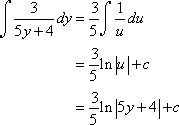# Welcome to Mathematics Help!

## What does this site cover?

This site covers certain topics from Calculus II. However, this site will only cover some basics about the course such as finding integrals, definite integrals, types of series, and others.
You will leave this site feeling satisfied and ready for your test! :)

## What is Calculus?

Calculus is a type of mathematics that involves finding the derivative of a function, finding the integral of a function and many other things. Usually, Calculus is known for it's limits (when a function approaches but never equates to a number), derivatives (the change of a function) and integrals (the opposite of a derivative).

## An Example of Calculus

This basic example will show you a sneak peak of what Calculus II is about. Here is the example:Find this a bit difficult? Don't worry! With practice, you'll get this with time! Now, let's get started!